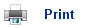# Mass Spectrometry

• The Mass Spectrometer is a useful machine to have in chemistry, as it allows much of the “wet” chemistry to be replaced by more accurate methods of analysis.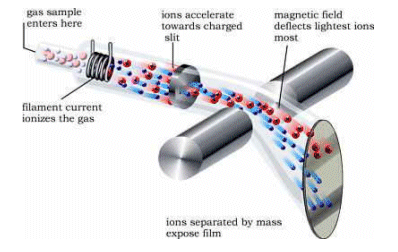• It is important that a low pressure is maintained in the spectrometer so that the ions can pass through unhindered by molecules in the air.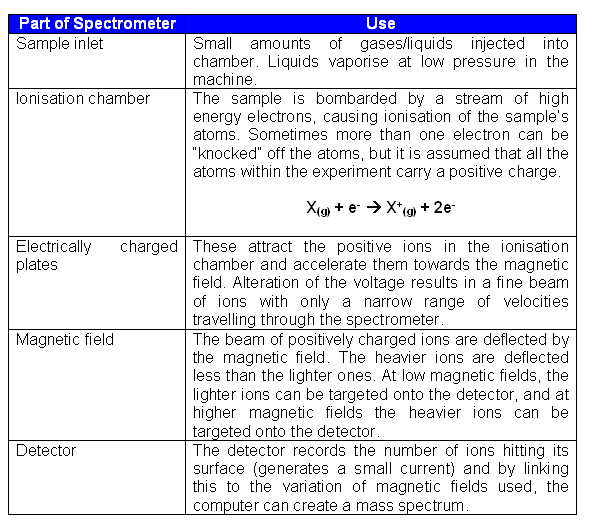## Fragmentation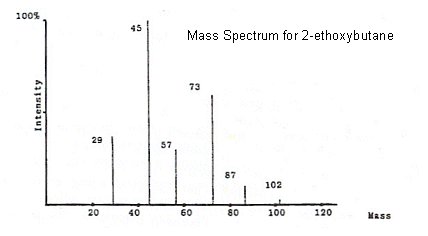• The molecular ion peak corresponds to the molecular mass of the compound being analysed. It is the peak furthest to the right on the spectrum.
• From the mass spectrum above, it is clearly evident that the ions which correspond to the molecular ion (X+) are not the only ones reaching the detector.
• This is because the other lines on the spectrum correspond to lighter ions that are the result of fragmentation (breakdown of X+).
• The strongest signal detected is set to 100% on the spectrum, and this is referred to as the base peak.
• The other peaks are expressed as a percentage fraction of the base peak's value.
• In some cases, the molecular ion peak can be unnoticeable as it is very small compared to the base peak.
• All of the peaks in 2-ethoxybutane spectrum can be identified. The mass of 2-ethoxybutane is 102, so if we look at the 87 peak for example, it has a mass 15 less than 2-ethoxybutane, and so it must have lost a CH3 group (CH3 has an Mr of 15).
• This process can be repeated with all of the peaks, for example: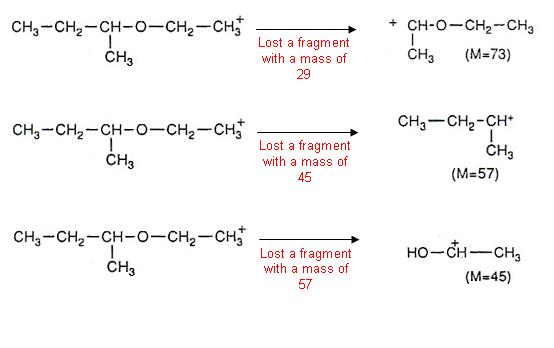• It is important to note that it is the positively charged fragments that are being measured by the mass spectrometer.
• Eventually the masses that have been lost from a compound become familiar. For example, 28 is the loss of a C=O group, 77 for the loss of a C6H5 (benzene ring) etc.

## Isotope Peaks

• Some elements have more than one naturally-occurring isotope. For example natural chlorine consists of 75% 35Cl and 25% 37Cl.
• So, what happens when a molecule containing chlorine is fragmented in the mass spectrometer? Well, there are two sorts of ions, one containing 35Cl and the other containing 37Cl.
• 35Cl will give a mass reading at M, and 37Cl will give a mass reading at M+2. The heights of the two peaks will be in ratio to the abundance of the isotopes, i.e. 3:1. A pair of peaks like this indicates that chlorine is present in the compound.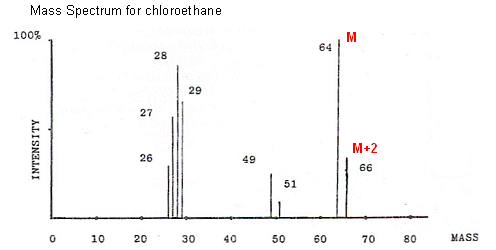• Another example of a useful isotope is Bromine. Bromine has an almost equal percentage of 79Br and 81Br isotopes; and so peaks that are the same height and 2 apart from each other indicate that Bromine is present in the molecule.
• 13C can be very useful for interpreting more complicated mass spectra. 13C accounts for around 1.1% of a sample of carbon atoms; however when there are many carbon atoms in a compound, the abundance of ions containing 13C will become more significant; the more carbon atoms in a molecule, the greater the chance of one of them being 13C.
• For example, decan-1-ol contains 10 carbon atoms, and so there will be an 11% chance (1.1% x 10) of at least one carbon atom being 13C, and so the molecular ion peak at 158 will be followed at 159 by a peak which is 11% as intense. This can be used to establish the number of carbon atoms in a compound.

## Useful books for revision

Revise A2 Chemistry for Salters (OCR A Level Chemistry B)Salters (OCR) Revise A2 ChemistryHome